# Explanation of Terms

 Output voltage This is the voltage measured at the energizer terminals, without the fence being connected. Voltage at a 500 Ohm load This is the voltage that you can measure at a fence under a normal load, e.g. animals or vegetation touching the fence. (= equals a load of 500 Ohm) Stored energy This is the true power of the energizer. You can compare it with the horse power of a engine. The stored energy value can be used to compare energizers with each other in a usefull way. Output energy This is the maximum energy that the enrgizer can put on to the fence. The higher the output energy, the higher the load can be (long fences, heavy vegetation on the fence etc.). Power Consumption The amount of energie a energizer uses is determined by the number of watts it uses. For example, a M1000 uses 10 watts per hour. This is much less than an average light bulb. 9 V Battery energizer uses a 9 Volt battery 12 V Battery energizer uses a 12 Volt battery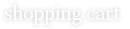•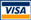•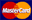•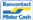Bucas

€21.95
Price: €19.76

Buzz-Off Reduction:
- 10%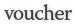Give a friend, aquaintance or family member a voucher for an amount of your choise.

more_info »

•••